# Java Program to Find the Largest Among Three Numbers

In this tutorial you will learn about the Java Program to Find the Largest Among Three Numbers and its application with practical example.

In this tutorial, we will learn to create a Java program that will find largest among three numbers  using Java programming.

## Prerequisites

Before starting with this tutorial we assume that you are best aware of the following Java programming topics:

• Java Operators.
• Basic Input and Output
• Class and Object.
• Basic Java programming.
• If-else Statements.

In Our Program, we going to learn how to creating a program that find the largest element between three numbers. Using nested if-else Statement.

## Java Program to Find the Largest Among Three Numbers

In this program we will find Largest among three .We would first declared and initialized the required variables. Next, we would prompt user to input three different values. Later we will find largest among them.

## Output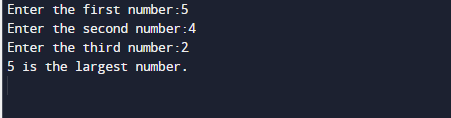In the above program, we have first declared and initialized a set variables required in the program.

• a = it will hold First value.
• b= it will hold second value.
• c= it will hold third value.

And  in the next statement user will be prompted to enter  different values  and  which will be assigned to variables a,b and c respectively.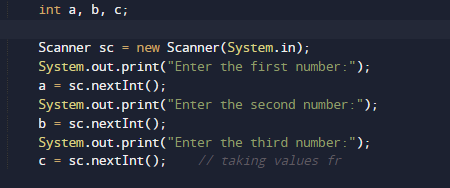Now we will check condition for greatest among three ,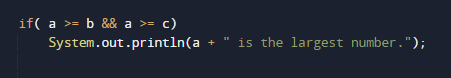If the above condition returns true value than the value in first variable is greatest among all the values.if it returns false value we will check  second condition for second variables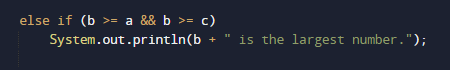this statement give true means second has the greatest among all value them and if gives false value we will move to next statement where we check the reaming third variable to other two if it give true i.e the value in third is greatest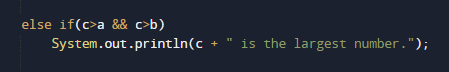else provided values is either equal of false.

In this tutorial we have learn about the Java Program to Find the Largest Among Three Numbers and its application with practical example. I hope you will like this tutorial.